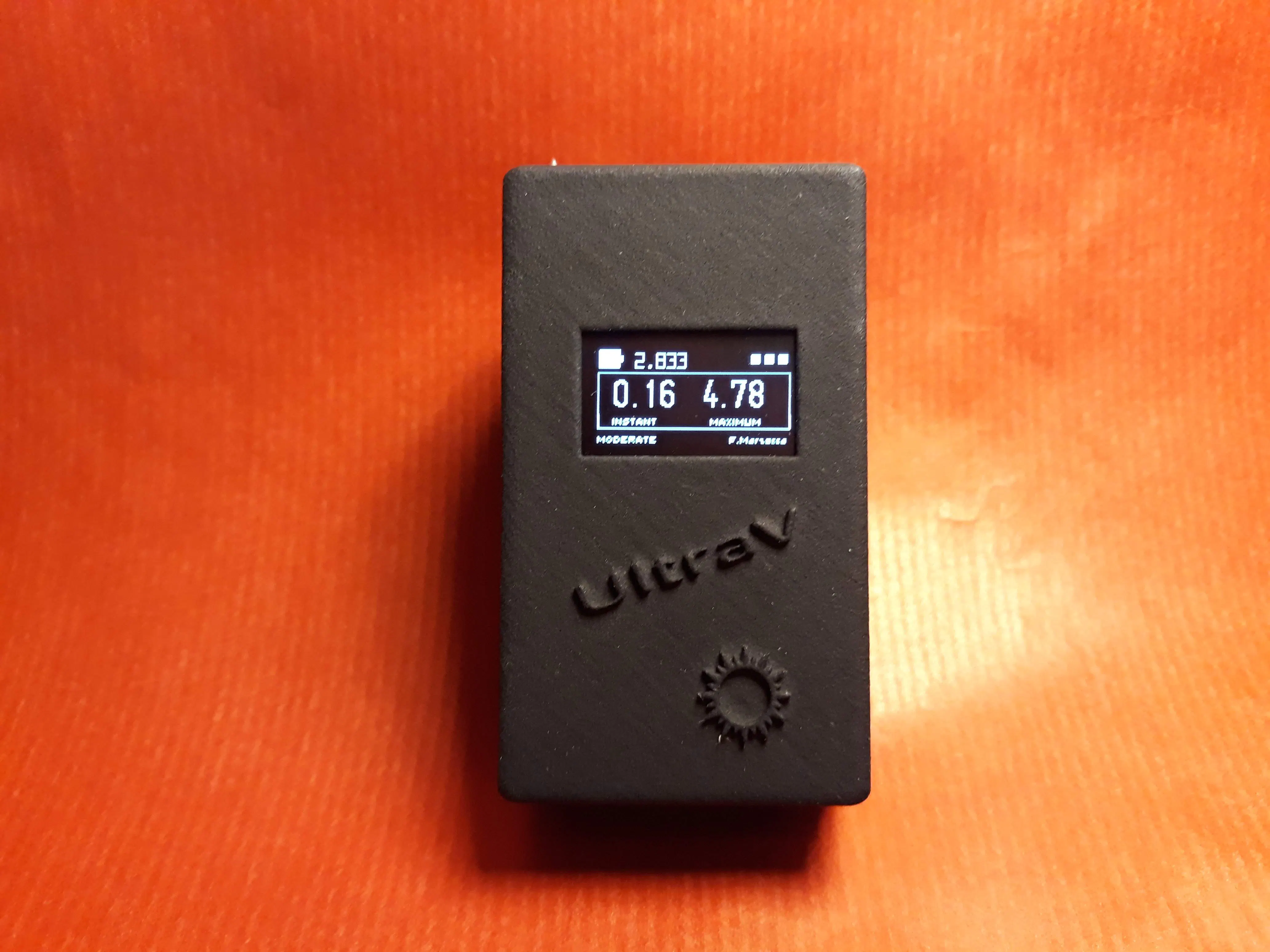# UltraV: A Portable UV-Index Meter

A portable small device to verify anywhere the UV index in the sun rays.

BeginnerFull instructions provided5,416## Things used in this project

### Hardware componentsArduino Nano R3
×1
 Texas Instruments ML-8511
×1
 128x64 OLED display
×1
 MT3608 DC-DC stup-up converter
×1
 CR2 (3v) battery
×1
 CR2 battery holder
×1

## Code

### UltraV

C/C++
Code
```    /*
Fabio Marzocca @ 2018
Analog to digital conversions rely completely on VCC. We assume
this is 5V but if the board is powered from USB this may be as high as 5.25V or as low as 4.75V:
http://en.wikipedia.org/wiki/USB#Power Because of this unknown window it makes the ADC fairly inaccurate
in most cases. To fix this, we use the very accurate onboard 3.3V reference (accurate within 1%). So by doing an
ADC on the 3.3V pin (A1) and then comparing this against the reading from the sensor we can extrapolate
a true-to-life reading no matter what VIN is (as long as it's above 3.4V).

v. 2.0.0 - July 2018
- moved from 16x2 LCD to OLED
v. 2.0.1 - Sept 2018
*/

#include <Arduino.h>
#include <U8g2lib.h>
#include <Wire.h>

U8G2_SSD1306_128X64_NONAME_F_SW_I2C u8g2(U8G2_R0, /* clock=*/ SCL, /* data=*/ SDA, /* reset=*/ U8X8_PIN_NONE);   // All Boards without Reset of the Display

#define FIRST_ROW_Y 16
#define FIRST_ROW_X 16
#define BOX_H 38

//Hardware pin definitions
const int UVOUT = A0; //Output from the sensor
const int REF_3V3 = A1; //3.3V power on the Arduino board
const int VBATT = A2; //Battery voltage

float maxUV = 0; //Max UV index read

void setup()
{
pinMode(UVOUT, INPUT);
pinMode(REF_3V3, INPUT);
pinMode(VBATT, INPUT);

u8g2.begin();

}

void loop()
{
u8g2.firstPage();
do {

//Use the 3.3V power pin as a reference to get a very accurate output value from sensor
float outputVoltage = 3.3 / refLevel * uvLevel;

float uvIntensity = mapfloat(outputVoltage, 0.99, 2.6, 0.0, 15.0); //Convert the voltage to a UV intensity level

if (maxUV < uvIntensity) {
maxUV = uvIntensity;
}
u8g2.drawFrame(0,FIRST_ROW_Y+1,128,BOX_H);
u8g2.setFont(u8g2_font_logisoso18_tf);

//Instant UV
u8g2.setCursor(10,40);
u8g2.print(uvIntensity);
//UV Max
u8g2.setCursor(70,40);
u8g2.print(maxUV);

u8g2.setFont(u8g2_font_u8glib_4_tf);
u8g2.setCursor(10,52); u8g2.print(F("INSTANT"));
u8g2.setCursor(75,52); u8g2.print(F("MAXIMUM"));

showUVCategory();
u8g2.setCursor(88,64); u8g2.print(F("F.Marzocca"));

} while ( u8g2.nextPage() );
delay(200);
}

// Reads maxUV and prints the UV category
void showUVCategory() {
char strCat;

byte categ = (byte)(maxUV+0.5);  //round up
if ((categ >= 0) && (categ < 3)) {
strcpy(strCat, "LOW");
} else if ((categ >= 3) && (categ < 6)) {
strcpy(strCat, "MODERATE");
} else if ((categ >= 6) && (categ < 8)) {
strcpy(strCat, "HIGH !");
} else if ((categ >= 8) && (categ < 10)) {
strcpy(strCat, "VERY HIGH!");
} else if (categ >= 11) {
strcpy(strCat, "EXTREME!");
}

u8g2.setCursor(0,64);
u8g2.print(strCat);

}

//Takes an average of readings on a given pin
//Returns the average
{
unsigned int runningValue = 0;

for(int x = 0 ; x < numberOfReadings ; x++)

return(runningValue);
}

//The Arduino Map function but for floats
//From: http://forum.arduino.cc/index.php?topic=3922.0
float mapfloat(float x, float in_min, float in_max, float out_min, float out_max)
{
return (x - in_min) * (out_max - out_min) / (in_max - in_min) + out_min;
}

long VccMin = 2300; //Battery minimum voltage read on Vcc
byte batteryBar; //Battery progress bar
int barStep = 140; //step for battery progress bar (235)

batteryBar = (battery - VccMin)/barStep;

//battery icon
u8g2.setFont(u8g2_font_open_iconic_embedded_2x_t);
u8g2.drawGlyph(1, FIRST_ROW_Y, 73 );

// battery status cursor
u8g2.setFont(u8g2_font_open_iconic_play_1x_t);
for (byte i=1; i<=batteryBar; i++) {
u8g2.drawGlyph( 128-9*i,FIRST_ROW_Y-4,75);
}

// battery voltage
u8g2.setFont(u8g2_font_freedoomr10_tu);
u8g2.setCursor(25, FIRST_ROW_Y);
u8g2.print(float(battery)/1000, 3);

}

{
int sum=0;
int sample_count=0;
long voltage= 0;
#define NUM_SAMPLES 10
// take a number of analog samples and add them up
while (sample_count < NUM_SAMPLES) {
sample_count++;
delay(2);
}
// calculate the voltage
// use 5000 for a 5.0V ADC reference voltage
// 5020V is the calibrated reference voltage (in millivolts) for my project
voltage = ((long)sum / (long)NUM_SAMPLES * 5020) / 1024.0;
return voltage; //Vbattery in millivolts
}

```